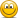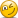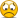# Market portfolio and derivative of weight?

#### Sergio Guerrero

##### New Member
Hi:

Is it possible to find out the standard deviation and expected return of the market portfolio by setting the first derivative of the Sharpe-ratio equal to “0”and solving for the weight of the first asset? And if so, is there an easier way? Thank you,I attached a word document because could not paste the formulaNote from Nicole: I added the image to your post for you @Sergio Guerrero#### Attachments

• market portfolio.pdf
52.1 KB · Views: 42
Last edited by a moderator:

#### Sergio Guerrero

##### New Member
I meant to say the tangent or risky portfolio, the one that sits on both the CML and the effcient frontier and has the best Sharpe-ratio. I was not sure if market portfolio means the same thing.

#### Sergio Guerrero

##### New Member
Hi:

Is it possible to find out the standard deviation and expected return of the market portfolio by setting the first derivative of the Sharpe-ratio equal to “0”and solving for the weight of the first asset? And if so, is there an easier way? Thank you,I attached a word document because could not paste the formulaNote from Nicole: I added the image to your post for you @Sergio GuerreroHi:

Is it possible to find out the standard deviation and expected return of the market portfolio by setting the first derivative of the Sharpe-ratio equal to “0”and solving for the weight of the first asset? And if so, is there an easier way? Thank you,I attached a word document because could not paste the formulaNote from Nicole: I added the image to your post for you @Sergio Guerrero#### Sergio Guerrero

##### New Member
Hi: I realized the formula Nicole was so kind to paste on my behalf did not make much sense by itself, so I tried to solve an example, which you will find attached, from Gruber & Elton, Reading7, page 21, but am not sure if my answer makes sense conceptualy. I set the first derivative of the Sharpe ratio equal to zero and solved for the weight of the first of the two assets of the tangent portfolio. Does this make sense? Thank you very much,

#### Attachments

• market portfolio.docx
11.4 KB · Views: 26

#### David Harper CFA FRM

##### David Harper CFA FRM
Staff member
Subscriber
@Sergio Guerrero your document merely sets up the problem, yes? (btw, i think you forgot to subtract the riskfree rate). I don't see any derivation attempt in the document ... if true, I have not seen such a solution, but when I get a chance, I will plug it into mathematica (this is ideal calculus prob for mathematica, imo).

#### QuantMan2318

##### Well-Known Member
Subscriber
I meant to say the tangent or risky portfolio, the one that sits on both the CML and the effcient frontier and has the best Sharpe-ratio. I was not sure if market portfolio means the same thing.

You are correct.
The tangency point on the efficient frontier where the CML touches is the Market Portfolio and that is the point where the Sharpe ratio is the highest

I am not sure if you can find the market portfolio that way because, the Sharpe ratio is in itself the slope of the efficient frontier and finding the derivative of the Sharpe ratio is like finding the second derivative of the efficient frontier.

Your solution intrigues me though, we can plot the Sharpe ratios of our portfolios vis a vis our Standard deviations and can find a maximum by setting the derivative of that curve equal to zero and finding out the corresponding SD, can we extend that to weights? I think so why don't you try it out on Excel?

The way I can think of finding the Market portfolio is to take a sample of portfolios, find their SD and Sharpe ratios and then use Solver to find the point where the Sharpe ratio is at its maximum.

I may be wrong also, but I am just contemplating

#### David Harper CFA FRM

##### David Harper CFA FRM
Staff member
Subscriber
@QuantMan2318 agreed about simulation: it's a trivial simulation problem (I'll show the R code when I get a chance) but @Sergio Guerrero is just asking: similar to how we solve for the weight (w) in the minimum variance portfolio by finding the local minimum of the portfolio variance function, can we assume the function for the sharpe ratio, take the first derivative (dS/dw; ie, with respect to weight), set it equal to zero, and solve for (w) to find the local maximum. The weight implied by solving for S'[w | rf, ....] = 0. Thanks,

Last edited:

#### Sergio Guerrero

##### New Member
Thank you: I attached my derivation attempt in a Word document in my prior Post. I tried to solve a problem on reading 7, page 21 I believe. The formula Nicole was kind enough to paste for me on my first post is not very useful and the whole procedure is missing.

#### Sergio Guerrero

##### New Member
Thank you very much David and Quantman! I went over the problem again and you are absolutely right David, I forgot to subtract the risk free rateI ran the numbers again and came up with a weight of 37.5% . Would Excel solver work for solving problems like this one?

#### David Harper CFA FRM

##### David Harper CFA FRM
Staff member
Subscriber
@Sergio Guerrero okay I see how you setup the problem by showing the sharpe ratio (if you subtract the riskfree rate) and then setting w' = 0 (w' does not actually equal the sharpe ratio), but I don't see even the first step in a "derivation" (sorry?). As mentioned, I'll run it in mathematica when i get a change. Re: Excel solver. Yes, for sure. That's what I did in the learning XLS. See https://www.dropbox.com/s/yc3j0g81m598tld/1.b.1. capm_v3.xlsx?dl=0 see tab 4.CML and then "Use Solver to Maximize Sharpe Ratio"

#### David Harper CFA FRM

##### David Harper CFA FRM
Staff member
Subscriber
@Sergio Guerrero and @QuantMan2318 Sure enough, amazing mathematica solves it (see below). Almost as amazing, I entered the solution into Excel and it seems to work perfectly given a few various test values (I got that formula in on the first try, i thought for sure i'd muck it up...). Tomorrow I'll get it into properly formatted into our learning spreadsheet and share it back for you to see. Thanks for asking Sergio, I am excited to have an analytical solution right in the spreadsheet, who knew!!!Last edited:

#### David Harper CFA FRM

##### David Harper CFA FRM
Staff member
Subscriber
@Nicole Seaman okay thanks, it is supposed to be a "public share" of the cloud-based notebook above, but I can't seem to get that working. Thanks!

#### Sergio Guerrero

##### New Member
@Sergio Guerrero and @QuantMan2318 Sure enough, amazing mathematica solves it (see below). Almost as amazing, I entered the solution into Excel and it seems to work perfectly given a few various test values (I got that formula in on the first try, i thought for sure i'd muck it up...). Tomorrow I'll get it into properly formatted into our learning spreadsheet and share it back for you to see. Thanks for asking Sergio, I am excited to have an analytical solution right in the spreadsheet, who knew!!!#### Sergio Guerrero

##### New Member
@Sergio Guerrero okay I see how you setup the problem by showing the sharpe ratio (if you subtract the riskfree rate) and then setting w' = 0 (w' does not actually equal the sharpe ratio), but I don't see even the first step in a "derivation" (sorry?). As mentioned, I'll run it in mathematica when i get a change. Re: Excel solver. Yes, for sure. That's what I did in the learning XLS. See https://www.dropbox.com/s/yc3j0g81m598tld/1.b.1. capm_v3.xlsx?dl=0 see tab 4.CML and then "Use Solver to Maximize Sharpe Ratio"
@Sergio Guerrero okay I see how you setup the problem by showing the sharpe ratio (if you subtract the riskfree rate) and then setting w' = 0 (w' does not actually equal the sharpe ratio), but I don't see even the first step in a "derivation" (sorry?). As mentioned, I'll run it in mathematica when i get a change. Re: Excel solver. Yes, for sure. That's what I did in the learning XLS. See https://www.dropbox.com/s/yc3j0g81m598tld/1.b.1. capm_v3.xlsx?dl=0 see tab 4.CML and then "Use Solver to Maximize Sharpe Ratio"

Hi: Is there by any chance a tutorial for solving this problem with "solver"? If not, I will try on my own and perhaps could get back to you should I have any doubts. I included this time a picture of my derivation attempt but just for the sake of formality. Thanks again for answering my question so thoroughly.

#### Sergio Guerrero

##### New Member
Dear David and Nicole:
I was able to find out the minimum variance and tangent portfolios using Excel Solver. Thank you for sharing the spreadsheet. This early in the course, should I be concerned with finding the tangent portfolio of many assets using Solver? Is this something we will learn later in the course?
Thank you very much,

#### David Harper CFA FRM

##### David Harper CFA FRM
Staff member
Subscriber
Hi @Sergio Guerrero Thanks! Re "should I be concerned with finding the tangent portfolio of many assets using Solver?" No, not for the exam. Aside from not being able to use Excel at the exam, more importantly, it is too tedious for the exam. The exam, if it does test on this topic, will be limited to a conceptual query (or, for example, calculating a Sharpe ratio given inputs). The exam is four hours, which implies an average limit of 2.4 minutes per Part 1 question (4*60/100) and 3.0 minutes per Part 2 question (4*60/80), so this is a key practical feature of the exam: questions cannot be too tedious and/or time-consuming. You can still see that we (bionicturtle) prefer to go deep on the concepts because (i) it encourages genuine mastery and (ii) we hope the concepts have a life (on the job) beyond the exam. Thanks!

#### agattik

##### New Member
I am having trouble reconciling the fact that the minimal variance portfolio has a positive quantity both A and B, with the formula for the optimal hedge ratio (Miller p.46), h* = −ρAB*σA/σB = -0.15, i.e. the minimal variance portfolio should short B, since A and B are positively correlated.